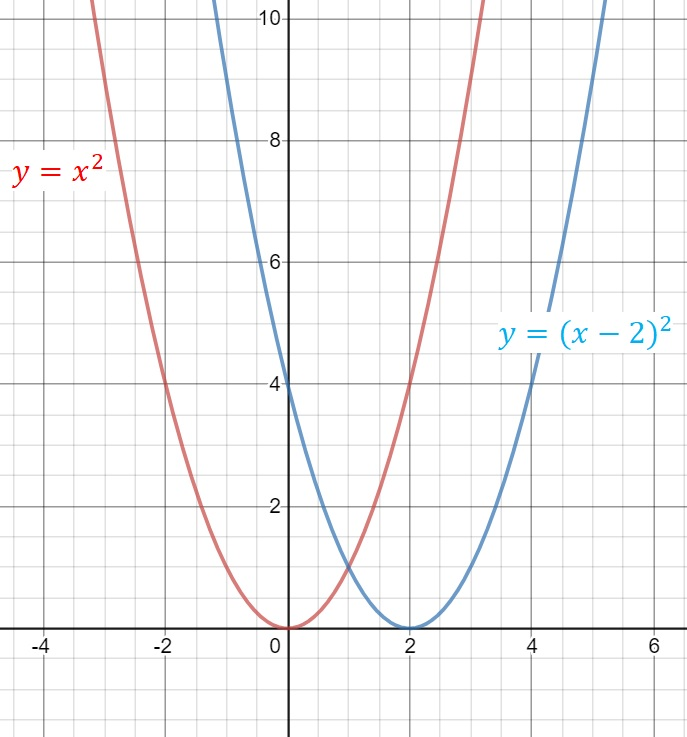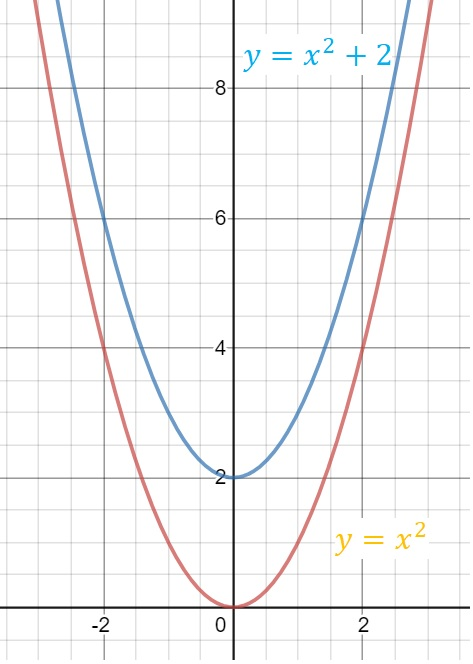# Notes – Transformation

HORIZONTAL TRANSLATION
A horizontal translation takes on the form  $f\left(x\right)\to f\left(x–a\right)$VERTICAL TRANSLATION
A vertical translation takes on the form  $f\left(x\right)\to f\left(x\right)+b$  .DILATION FROM THE  $x–axis$

For the curve with equation  $y=pf\left(x\right)$  ,
$\left|p\right|>1$ , represents ‘stretching’  $f\left(x\right)$ by a factor $p$ from the $x$ –axis.
$0<\left|p\right|<1$  , represents ‘shrinking’ $f\left(x\right)$ by a factor  $\frac{1}{p}$ from the $x$ –axis

DILATION FROM THE $y–axis$

For the curve with equation  $y=f\left(\frac{x}{q}\right)$ ,
$\left|q\right|>1$ , represents ‘stretching’ $f\left(x\right)$ by a factor  $q$ from the $y$ –axis.
$0<\left|q\right|<1$  , represents ‘shrinking’ $f\left(x\right)$ by a factor  $\frac{1}{q}$ from the $y$ –axis.